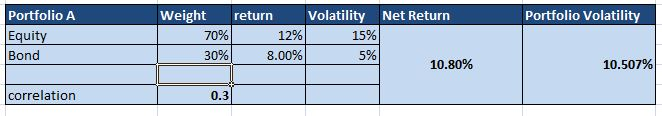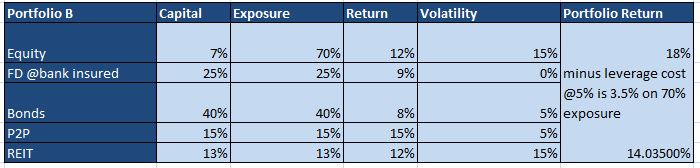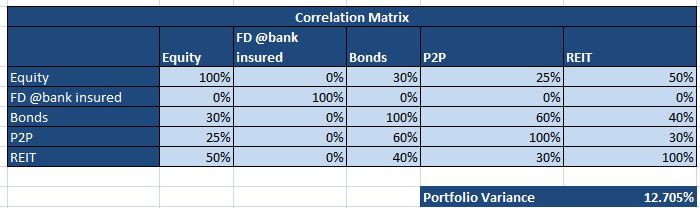# Portfolio Diversification and Alternate Investment

It is known fact that diversification helps in reduction of total risk . Conventional portfolio use this principle and diversify between bonds and equity etc. This is the foundation of modern portfolio Theory.

Let’s take an example . Mr X is 30 year old. Conventional wisdom say he should invest 70% in equity and 30% in Debt.

Let’s look at the risk and return parameters of this portfolio.

let’s assume Nifty gives annual return of 12% in the long run .Historical volatility of Nifty is around 15% per annum.

let the debt portfolio gives 7.5% with annual volatility of 5%.

so a portfolio with 70% in equity and 30% in debt will give = 70% * 12% + 7.5%*30% =10.8%

Portfolio volatility is calculated asPortfolio Volatility = 10.5% for our portfolio

almost 85% volatility is attributed from the equity portfolio which makes the return quite unstable with frequent drawdown.

In contrast to this another portfolio allocation is in practice where you try to balance the risk(volatility) by leveraging low volatility asset class. It’s called Risk Parity

Risk Parity: Risk Parity is approach where portfolio weights are created according to equal contribution of risk. It implies that you can  take leverage for bonds and lower contribution of equity so that your output in terms of return is such that both  equity  and bonds contribute equal  to  volatility.

There is another approach which can be followed which lies somewhere in between and provides good risk adjusted return.

Instead of 70% Equity and 30% bond you will have 30% equity and 130% bond etc so your return matches equity but volatility is low.Problem is during a equity bull run it will under perform the other portfolio

I try to create a portfolio which lies in between these 2 methods where I can participate in equity and also lower my volatility to certain extent. The idea is to find very low correlated assets.

Let’s compare this portfolio (Portfolio B) with traditional Portfolio(Portfolio A):

70% Equity 30%  BondI have considered correlation between bonds and equity as 0.3 based on some market reports. It would vary depending on types of bond and historical period you take. Idea is to show the correlation is low.

Portfolio B: Hybridbasically I have used futures instead of stocks to take equity exposure( I am able to leverage 10X approx i.e. by putting 7% margin I get exposure to 70% portfolio.

Cost of exposure is the future premium which is around 5%.

Instead of using future I can also use combination of options. You need to be proficient in Option Trading to implement that strategy

My Returns are higher compared to traditional portfolio because I have leverage. Important part is how much is my portfolio volatility.

I have taken correlation from various reports and true numbers can deviate from my numbers. Essence is to show that most of these assets are not strongly correlated as they have different underlying viz equity, credit, Real estate, Retail loans, Bank FD

Correlation for FD portfolio is zero as it wont show any deviation. I have posted article on creating risk free FD portfolio.

http://randomdimes.com/2019/create-a-risk-free-fixed-deposit-portfolio/

How to calculate Portfolio Variance for multiple assets with correlation?

Here is the formula:Based on this my Portfolio Standard deviation isMy new portfolio has better risk adjusted return i.e for lower volatility I am achieving higher return. Offcourse you need to monitor this portfolio for margin requirement,re balancing etc.

If someone is very conservative they can further increase FD exposure ,reduce equity and portfolio volatility will drop further at the cost of some returns!!